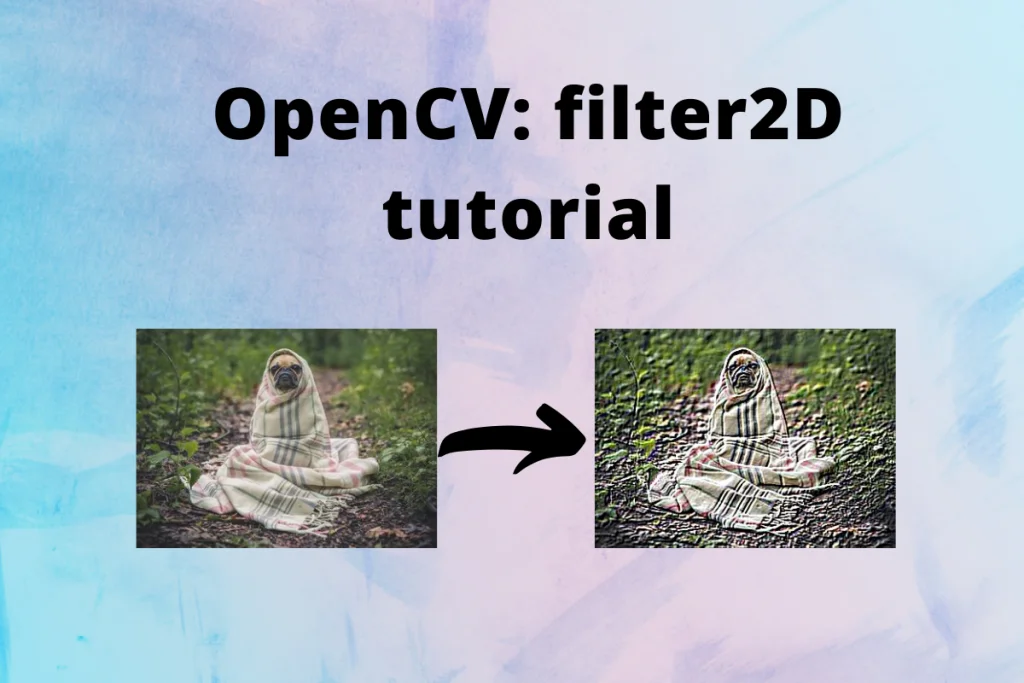# Python OpenCV filter2D() function – A Complete GuideHello everyone! In this tutorial, we will learn how to use OpenCV `filter2D()` method to apply filters on images such as sharpening, bluring and finding edges in the images. So lets get started.

## Introduction to the OpenCV filter2D() function

While dealing with images in Image Processing, `filter2D()` function is used to change the pixel intensity value of an image based on the surrounding pixel intensity values. This method can enhance or remove certain features of an image to create a new image.

Syntax to define `filter2D()` function in python is as follows:

```resulting_image = cv2.filter2D(src, ddepth, kernel)
```
• src: The source image on which to apply the fitler. It is a matrix that represents the image in pixel intensity values.
• ddepth: It is the desirable depth of destination image. Value -1 represents that the resulting image will have same depth as the source image.
• kernel: kernel is the filter matrix applied on the image.

More formally, `filter2D()` function convolves an image with the kernel which results in an image becoming blur or sharpen and enhances the image features.

### What is a kernel?

Also known as convolution matrix or mask, kernel is a small 2-dimensional matrix containing values that represent how much part of surrounding pixel values it should take to calculate intensity value of the current pixel. Usually, kernels are square matrices of odd length like 3×3, 5×5, 7×7 matrices.

Thus, the kernel act as a weighted matrix and is used for the blurring of images, sharpening of images, detection of edges in the images, etc. in image processing. This is done by convolution between image and kernel.

### What is Convolution?

In Image Processing, Convolution is simply an element wise multiplication of kernel and some part of source image to produce a new single data point representing a pixel, doing it on every possible part of image to create a new image.

In Convolution, we take a submatrix from source image of same size as that of kernel, multiply each element of source image with corresponding element of kernel, perform addition on the previous computation and normalize the data so as to represent the data as pixel value.

Consider an example as shown in the image below:

Convolution on an image can result in an image of size less than the source image. The difference depends on the size of our kernel. However, there are ways to deal with it as discussed here.

## Using OpenCV filter2d() with different kernels

Let’s apply the `filter2d()` function on an image with different kernels and see what results we get. For this example, we will use the following image.

### 1. Sharpening an Image

```import cv2
import numpy as np
# Defining the kernel of size 3x3
kernel = np.array([
[0, -1, 0],
[-1, 5, -1],
[0, -1, 0]
])

resulting_image = cv2.filter2D(src_image, -1, kernel)

cv2.imshow("original image", src_image)
cv2.imshow("filter2d image", resulting_image)
cv2.imwrite("Filter2d Sharpened Image.jpg", resulting_image)
cv2.waitKey()
cv2.destroyAllWindows()
```

### 2. Bluring an image

```import cv2
import numpy as np
# Defining the kernel of size 3x3
kernel = np.array([
[1, 1, 1],
[1, 1, 1],
[1, 1, 1]
]) / 9

resulting_image = cv2.filter2D(src_image, -1, kernel)

cv2.imshow("original image", src_image)
cv2.imshow("filter2d image", resulting_image)
cv2.imwrite("Filter2d Blur Image.jpg", resulting_image)
cv2.waitKey()
cv2.destroyAllWindows()
```

### 3. Outline Edge Detection on an image

Let’s look at edge detection with the OpenCV filter2D() function.

```import cv2
import numpy as np
# Defining the kernel of size 3x3
kernel = np.array([
[-1, -1, -1],
[-1, 8, -1],
[-1, -1, -1]
])

resulting_image = cv2.filter2D(src_image, -1, kernel)

cv2.imshow("original image", src_image)
cv2.imshow("filter2d image", resulting_image)
cv2.imwrite("Filter2d Outline Image.jpg", resulting_image)
cv2.waitKey()
cv2.destroyAllWindows()
```

### Using Emboss Filter

```import cv2
import numpy as np
# Defining the Emboss kernel of size 3x3
kernel = np.array([
[-2, -1, 0],
[-1, 1, 1],
[0, 1, 2]
])

resulting_image = cv2.filter2D(src_image, -1, kernel)

cv2.imshow("original image", src_image)
cv2.imshow("filter2d image", resulting_image)
cv2.imwrite("Filter2d Emboss Image.jpg", resulting_image)
cv2.waitKey()
cv2.destroyAllWindows()
```

### Using Sobel Filter

```import cv2
import numpy as np
# Defining the Sobel kernel of size 3x3
kernel = np.array([
[-1, 0, 1],
[-2, 0, 2],
[-1, 0, 1]
])

resulting_image = cv2.filter2D(src_image, -1, kernel)

cv2.imshow("original image", src_image)
cv2.imshow("filter2d image", resulting_image)
cv2.imwrite("Filter2d Sobel Image.jpg", resulting_image)
cv2.waitKey()
cv2.destroyAllWindows()
```